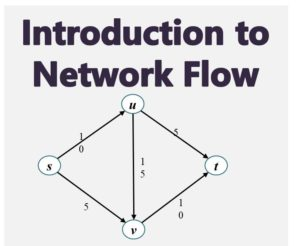# Introduction to Network Flow1. Introduction to Network Flow

Networks are often used in various areas of real life application. These networks when operational, usually carry some some sort of traffic. For example:
Transportation Network – traffic of vehicles

• Computer Network  – traffic of data packets
• Electrical Networks – traffic of electrical signals
• City Water Board – traffic of water
• etc

All these can be model using graphs with nodes and edges. Consider for instance, the transport system where the edges represents the highways and the nodes represents interchanges; or a computer network where the nodes are the switches (hubs, routers, bridges) and the edges are the links.

These kinds of network have something is common, and that  is:

• Capacity on the edges: how much traffic the edge can carry
• Source:  the points(nodes) where the traffic is generated
• Sink: the points (nodes) where the traffic is terminated.

The source is said to originated the traffic while the sink absorbs it.

2. What is Flow Network?

Flow networks is a graph used to model the systems described in the introduction. Here, the traffic is called a flow, which is transmitted across from the source node through the edges and nodes to the sink node.

A flow network is  a directed graph given a G(V, E) with the following characteristics:

• Each edge has a capacity which is denoted by ce
• There is a single node which is the source represented as s ∈ V
• there is a single sink node represented as t ∈ V

Nodes that are neither t or s are known as internal nodes. An example of a flow network in given in Figure 1 where the numbers besides the edges represents the capacity of the edge.

To be able to analyse flow networks, we would make the following two assumptions:

• there is no edge entering the source s and there is not edge leaving the sink t
• there is at lease one edge (entering or leaving) each node
• all capacities of the edges are non-negative integers.

These are shown in the network of Figure 1. Lets now go ahead to define a flow.

3. What is a Flow?
Now, we want to try to define the traffic carried by the network.

An s-t flow is a function f that maps each edge e of the network to a non-negative number, and given by f: E → R+.  The value of f represents the flow, which is the amount of traffic currently carried by each edge. A flow f must satisfy the following constraints:

(a) Capacity Constraint: For each edge e ∈ E,  0 ≤ f(e) ≤  ce

(b) Conservation Constraint: For each node v that is not s or t, we have:

∑ f(e into v)  = ∑ f(e out of v)

This means that the sum of the flows through all the nodes entering into node v equals the sum of the flows through all the nodes leaving node v. This also means that the flow on an edge cannot exceed the capacity of the edge.

The value of of a flow f is denoted as v(f) is defined as the volume of flow generated at the source. This is give as

v( f) =  f(e out of the source)

Also for the sink, we have

v( f) =  f(into the sink)

We can further simplify by defining the following for all the nodes in the network:

fout(v) = ∑e out of v f(e)

In the same way, we can defined the flow entering a vertex c

fin(v) = ∑e into v f(e)

By now you can realize that for each of the internal nodes v, :

fout(v) = fin(v)

For the source node S, we have: fout(s) = ∑e out of s f(e) which gives us the value of the flow v(f) =  fout(s)

4. Maximum Flow Problem.

The maximum flow problem is that of finding the combination of flows that would make the most efficient use of the capacity of the network.  We attempt to formulate this problem this way:

assuming we partition the nodes of the network into two sets: A and B such that s  A and t    B. Then any flow that goes from s to t has to cross from A to B, using up the edge capacity from A to B. This would mean that such a cut would put a bound on the maximum possible flow value of the network.

The maximum flow value(max-flow) would equal the minimum capacity of any such cut (min-cut). We would examine details of this in the next article.#### kindsonthegenius

Kindson Munonye is currently completing his doctoral program in Software Engineering in Budapest University of Technology and Economics

View all posts by kindsonthegenius →